Create a new printable
Answer key also includes questions
Answer key only gives the answers
No answer keyMath Worksheets

Sample - Click above to make a new math worksheet (PDF).
 Name _____________________________Date ___________________
Subtraction and Addition
Subtract and then add to check your answer.

1.
What is 22 - 8?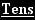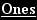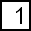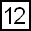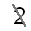- 8 1 4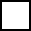+
2.
What is 83 - 7?8 3 - 7+
 3 * This is a pre-made sheet.Use the link at the top of the page for a printable page.
4.
What is 12 - 3?1 2 - 3+
5.
What is 51 - 6?5 1 - 6+
6.
What is 47 - 9?4 7 - 9+

 Answer Key

Sample
This is only a sample worksheet.

Create a new printable
Answer key also includes questions
Answer key only gives the answers
No answer keyMath Worksheets